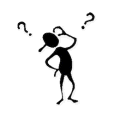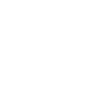## Special...23 is the smallest odd prime which is not a twin prime.

is one of only two positive integers which CANNOT be written as the sum of 8 positive cubes (239 is the other one).
--- Christopher Carl Heckmann (23 letters!)

is the smallest prime number with consecutive digits.
is the lowest prime whose reversal is a power: 32 = 25 - Trigg
is the lowest number of integer-sided parallelepipeds you can fit in a box so that no two parallelepipeds share a common length.
occurs twice in the list of the fortunate numbers.

Palindromic equalities involving 23:
23 x 64 = 46 x 32
23 x 96 = 69 x 32

23! is 23 digits long.
= 5 + 7 + 11 (smallest prime that is a sum of 3 consecutive primes)
= (3 x 5) + (3 + 5)
= 3 x 2³ + (-1)³ (sum of 4 cubes)
= 2 x 2³ + 7 x 1³ (sum of 9 positive cubes)
= 12² - 11²
= - (2² - 3³)
= 14 + 2³ + 3² + 41 + 50

1023 - 23 = 99,999,999,999,999,999,999,977 is the largest 23 digit prime.

23 x 2239 x 2393 = 123232321 (palindromic prime)

Repeat the digit 1, 23 times like this: 11,111,111,111,111,111,111,111 and you obtain a prime number.

23.140692632779269005... is eð, also known as the Gelfond's constant.

The 'Birthday Paradox' states that a group of 23 randomly-selected persons is the lowest number where there will be a probability higher than 50 per cent that two people will share the same birthday. For 60 or more people, the probability is greater than 99 per cent!

In telegraphers code 23 means "break the line".

Each parent contributes 23 chromosomes to the start of human life. The nuclei of cells in human bodies have 46 chromosomes made out of 23 pairs.

Twenty-three is the Sacred Number (along with 5) of Eris, goddess of discord, according to the Principia Discordia; it is the number of the 'Illuminati'.

Twenty-three is one of the most commonly cited prime numbers... Numerous people have claimed to see 23's everywhere (the '23 curse') prior to enormous success and fruitfulness. 23rdians are a group of persons who subscribe to the mystical power of 23 and see it in multiple combinations throughout daily life.

The 23rd letter of the alphabet is 'W'. Therefore, white supremacists and racist skinheads use 23 in tattoos to represent 'W', as an abbreviation for the word "white".

The Earth is tilted at an angle of 23.44 degrees (at the moment!).